﻿ Analytical and FDM Solutions for Anisotropic Heat Conduction in an Orthotropic Rectangular

### Analytical and FDM Solutions for Anisotropic Heat Conduction in an Orthotropic Rectangular

Mohammad Sameti, Fatemeh Razi Astaraie, Fathollah Pourfayaz, Alibakhsh KasaeianOPEN ACCESSPEER-REVIEWED

## Analytical and FDM Solutions for Anisotropic Heat Conduction in an Orthotropic Rectangular

Mohammad Sameti1, Fatemeh Razi Astaraie1, Fathollah Pourfayaz1,, Alibakhsh Kasaeian1

1Department of Renewable Energies, Faculty of New Sciences & Technologies, University of Tehran, Tehran, Iran

### Abstract

Anisotropic materials have physical properties such as thermal conductivity which vary with orientation throughout the structure. These properties make the analysis of heat transfer much more complex than standard isotropic materials. Therefore, the heat flux equation and Fourier’s Law should be expanded in order to properly govern the thermal conduction. The analytical exact solutions and numerical finite difference solutions of a fundamental heat conduction problem in an anisotropic medium are presented in this study. The problem is solved for steady state condition in a rectangular thin film of quartz. The key for analytical solution is to use two variables to convert the anisotropic equations into isotropic ones. The temperatures of 35 points on the rectangular are obtained and compared by the two methods. The results show an excellent accuracy for the numerical method. The temperature and the heat flux profile are also illustrated to show how the anisotropicity influences the thermal behavior of the media. MAPLE and EES were used to numerically integrate or solve the system of the eqautions.

### At a glance: Figures

12
Prev Next

• Sameti, Mohammad, et al. "Analytical and FDM Solutions for Anisotropic Heat Conduction in an Orthotropic Rectangular." American Journal of Numerical Analysis 2.2 (2014): 65-68.
• Sameti, M. , Astaraie, F. R. , Pourfayaz, F. , & Kasaeian, A. (2014). Analytical and FDM Solutions for Anisotropic Heat Conduction in an Orthotropic Rectangular. American Journal of Numerical Analysis, 2(2), 65-68.
• Sameti, Mohammad, Fatemeh Razi Astaraie, Fathollah Pourfayaz, and Alibakhsh Kasaeian. "Analytical and FDM Solutions for Anisotropic Heat Conduction in an Orthotropic Rectangular." American Journal of Numerical Analysis 2, no. 2 (2014): 65-68.

 Import into BibTeX Import into EndNote Import into RefMan Import into RefWorks

### 1. Introduction

There are many natural and synthetic materials in which the thermal conductivity varies with direction and are called anisotropic materials. For example, crystals, wood, sedimentary rocks, metals that have undergone heavy cold pressing, laminated sheets, cables, heat shielding materials for space vehicles, fiber reinforced composite structures and many others are anisotropic materials. Ma and Chang  considered a two-dimensional steady state thermal conduction problem of anisotropic multi-layered media. They used the Fourier transform and the series expansion technique to find the explicit closed form solutions of the problem. Maruyama et al. investigated the anisotropic heat transfer in a single-wall carbon nanotube using molecular dynamic simulation . Mera et al. solved the steady state anisotropic heat conduction problems by the implementation of the boundary element method (BEM) . Sladek et al. used the meshless methods based on the Petrov-Galerkin approach to solve the steady and transient heat conduction problem in a continuously nonhomogeneous anisotropic medium . Materials used for renewable heating and cooling of buildings can also have the anisotropic behavior .

In this study the steady state heat conduction is of interest and the finite difference method is implemented as the numerical solution. Two methods were combined to solve the problem, analytically. Then, the energy balance formula is used as in to numerically model the equation with finite difference method. In rectangular coordinates for two dimensional conduction, the magnitudes of heat flux qx and qy in the x and y directions can be determined from Fourier’s low: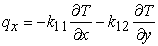(1)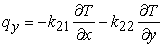(2)

Where T is the temperature and kij is the thermal conductivity. From thermodynamics, the determinant and the elements of the matrix M defined as: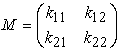(3)

Should be positive. This known as Onsager’s reciprocity relation .

### 2. Formulation of the Problem

A rectangular thin film which is made of quartz is considered in this study. As Figure 1 shows, owing to its low height l, instead of solving the three dimensional problem, the problem is assumed to be two dimensional. Temperature variations of the medium is negligible in the z direction, however it varies in the directions of x and y with different rates. The thermal and geometric specifications of the rectangular can be found in Table 1. The problem can be expressed as follows:

Figure 1. Orthotropic rectangular considered for the anisotropic heat conduction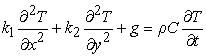(4)

Where g is the heat generation, ρ is the density and C is the specific heat. For steady state condition with no heat source, the equation is simplified to: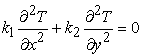(5)

Where 0<x<6 and 0<y<4. The boundary conditions for the temperature distribution on the sides are given by: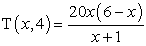(6)(7)
2.1. Analytical Solution

The heat conduction equation for an orthotropic medium can be transformed to a standard heat conduction equation for an isotropic medium . New independent variables X and Y are defined as: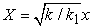(8)(9)

Where k is a reference conductivity. Equation (1) then becomes: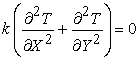(10)

The choice of the reference conductivity is not arbitrary. The reason for this is that a volume element in the original space dxdy transforms using equations (8) and (9) into equation (11):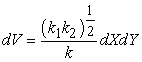(11)

If the quantities ρCp and the generation term g defined on the basis of unit volume should have the same physical significance, we should have:(12)

Now, the equation (10) which is the 2D potential equation can be solved using the separation of variables. The method is well-known and applicable for such problems . Assuming:(13)

Where T1(x) and T2(y) are independent functions. By introducing equation (13) into equation (10), it is obtained that: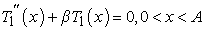(14)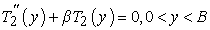(15)

Whereis the second derivative of the function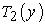. The boundary conditions then become: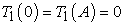(16)(17)

The length a and the width a of the rectangular is transformed to A and B, respectively.(18)(19)

Solving equation (14), it is obtained that the problem is the generator for the sinusoidal Fourier series where the eigenfunctions and eigenvalues are: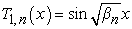(20)(21)

Where n is a natural number. Substituting equation (20) in equation (15) and solving it with the condition (17), yields: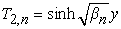(22)

Therefore, for each particular number n, the solution for the temperature is:(23)

The final answer is the summation of al, so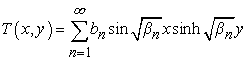(24)

The boundary condition (6) should now be used, but it is necessary to transform it to a new function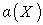using equations (8) and (9):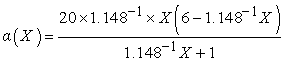(25)

Applying the boundary condition we have:(26)

Which is solved to find the Fourier coefficients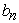as:(27)

#### Table 1. Specifications of the orthotropic media

The temperature at each point can now be found using MAPLE to numerically integrate equation (27) and to find the series answer in equation (26). Furthermore, the temperature profile and the heat flux profiles can also be illustrated.

2.2. Numerical Solution

A mesh can be made with 35 nodes in which the horizontal step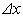and the vertical step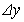are both equal to 1 cm. The grid is illustrated in Figure 2. In a steady state condition, the energy balance can be written for each of the nodes . For example, for the node number 9 the suitable equation is:(28)

The general equation for all of the nodes can be written as follows: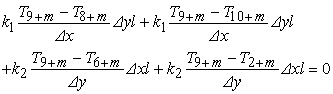(29)

Where m falls within the following interval.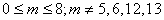(30)

For the other nodes, the temperature is given in equations (2) and (3) as:(31)

Now, 15 equations are created which can be solved using the software EES (Engineering Equation Solver).

### 3. Results and Discussions

The temperature for the 35 nodes is calculated by the both methods and can be found in Table 2. As it can be seen, the results are very similar and the numerical method is accurate enough. The isothermal curves and the heat flux vectors are also illustrated in Figure 3 and Figure 4. It can be understood from the results that:

1. The heat flux vectors are not perpendicular to isothermal curves (despite the isotropic heat conduction), because the heat flows in an anisotropic way in the medium.

2. The slope of all vectors is more than 1 or the angles are more than 45°. This happens because the conductivity in the horizontal direction is less than the vertical direction.

3. The variations in the surface temperature in equation (2) have led to the accumulation of isothermal curves in the left side and top of the rectangular (edge effects).

4. The temperature difference between the two methods reach its maximum level at node 9, because the surface temperature described by the equation (2) suddenly increases from 0 to 50 in that region.

### 4. Conclusions

Mathematically, it is understood that the most important difference between the isotropic and anisotropic heat conduction is that the heat flux vectors are not perpendicular to isothermal curves. Steady state anisotropic heat conduction problem is solved numerically and analytically for an orthotropic rectangular thin film. The analytical method is based on the introducing two new variables to transform the equations to the well-known 2D potential equation which can be solved by the separation of variables. The numerical method is based on the energy balance and the finite difference method. The results are compared and the results show an excellent accuracy.

### References

  Ma, C. C., & Chang, S. W. (2004). Analytical exact solutions of heat conduction problems for anisotropic multi-layered media. International journal of heat and mass transfer, 47 (8), 1643-1655.In article CrossRef  Maruyama, S., Igarashi, Y., Taniguchi, Y., & Shiomi, J. (2006). Anisotropic heat transfer of single-walled carbon nanotubes. Journal of Thermal Science and Technology, 1, 138-148.In article CrossRef  Mera, N. S., Elliott, L., Ingham, D. B., & Lesnic, D. (2001). A comparison of boundary element method formulations for steady state anisotropic heat conduction problems. Engineering analysis with boundary elements, 25 (2), 115-128.In article CrossRef  Sladek, J., Sladek, V., & Atluri, S. N. (2004). Meshless local Petrov-Galerkin method for heat conduction problem in an anisotropic medium. Computer Modeling in Engineering and Sciences, 6, 309-318.In article  Kasaeian, A., Sameti, M., & Eshghi, A. T. (2014). Simplified Method for Night Sky Radiation Analysis in a Cool-Pool System. Science and Education, 2(1), 29-34.In article  Ozisik, N. (1993). Heat conduction. John Wiley & Sons.In article  Su, S., Chen, J., & Zhang, C. (2011). Study on Performance of Anisotropic Materials of Thermal Conductivity. Open Civil Engineering Journal, 5.In article CrossRef  Kreyszig, E. (2010). Advanced engineering mathematics. John Wiley & Sons.In article  Incropera, F. P., Lavine, A. S., Bergman, T. L., & DeWitt, D. P. (2013). Principles of heat and mass transfer. Wiley.In article